Science, Maths & Technology

### Become an OU student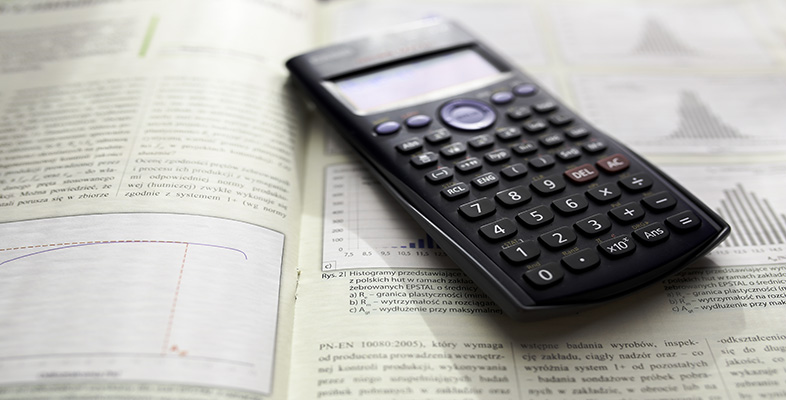Using a scientific calculator

Start this free course now. Just create an account and sign in. Enrol and complete the course for a free statement of participation or digital badge if available.

Your calculator can be set to calculate trigonometric functions using the radian measure for angles, instead of degrees, by using the key sequence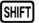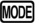(SETUP)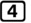(Rad).

When in this mode, the display indicator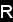is shown.

In this activity, the angles are measured in radians. Find the values of the following expressions, giving your answers correct to 3 significant figures.

1.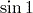2.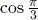3.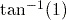1.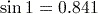(to 3 significant figures).
2.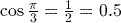.
Remember: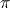can be input to the calculator using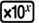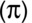.
3.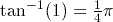or 0.785 (to 3 significant figures).
Notice from the final example in this activity that where an answer is a simple (possibly fractional) multiple of, the answer is displayed in terms ofrather than as a decimal number.